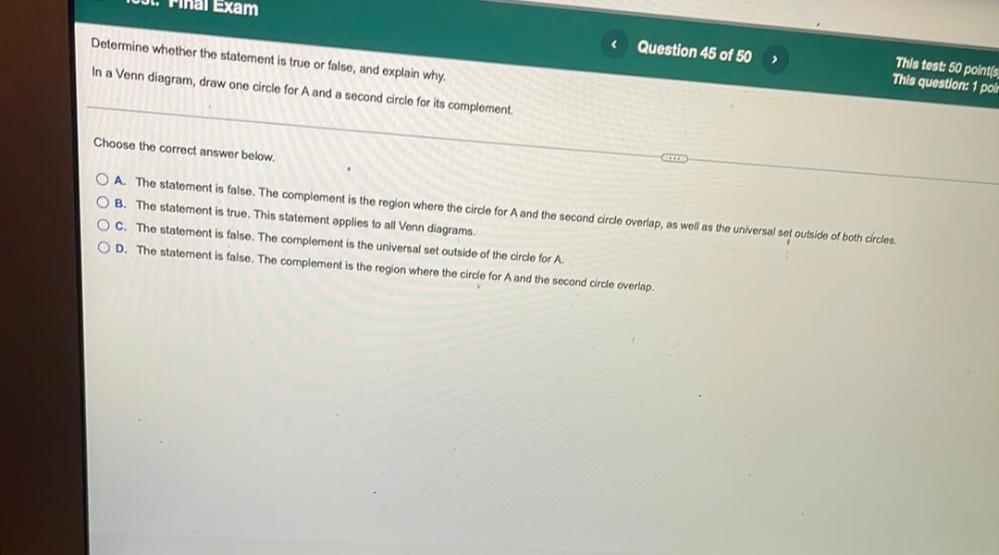Question:

# Exam Determine whether the statement is true or false, and explain why. < Question 45 of 50 In a Venn diagram, draw one circle fExam Determine whether the statement is true or false, and explain why. < Question 45 of 50 In a Venn diagram, draw one circle for A and a second circle for its complement This test: 50 polnt(s. This question: 1 polr Choose the correct answer below. O A. The statement is false. The complement is the region where the circle for A and the second circle overlap, as well as the universal sot outside of both circles. OB. The statement is true. This statement applies to all Venn diagrams. OC. The statement is false. The complement is the universal set outside of the circle for A. OD. The statement is false. The complement is the region where the circle for A and the second circle overlap.